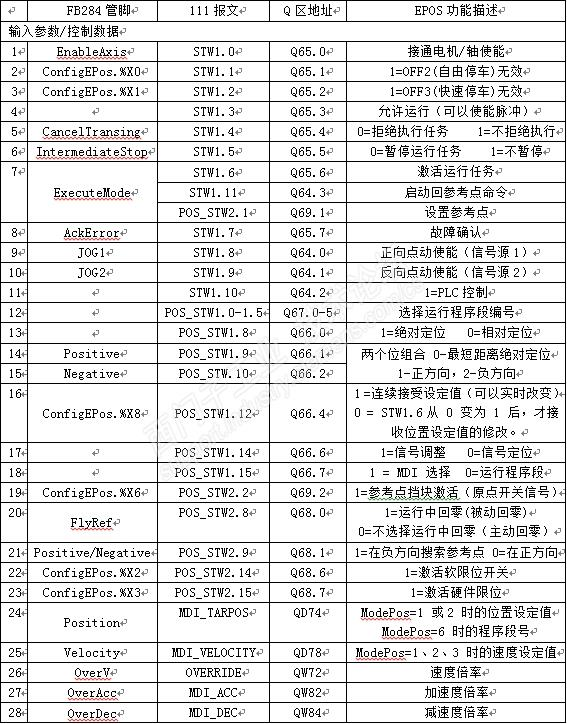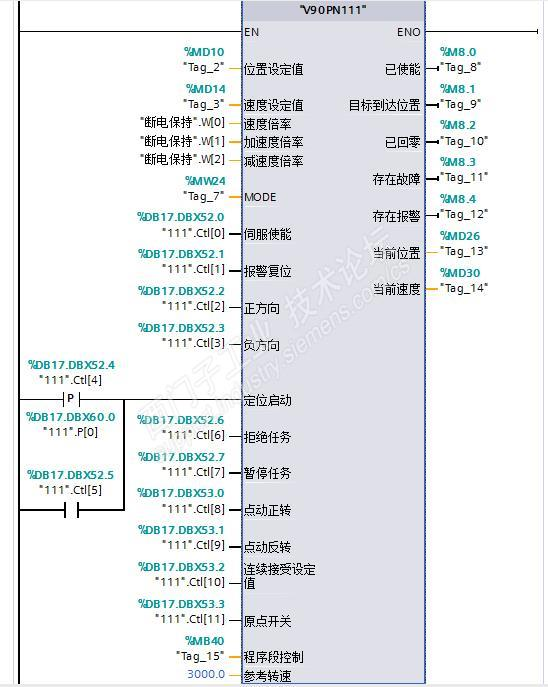# 技术论坛

• 产品分类
• 咱工程师的故事
• 培训与认证答疑专区
• 服务合作伙伴专区

###简单梳理下EPOS和FB284EPOS（基本位置控制）是位置环在驱动器侧，由驱动器自身完成位置闭环控制的功能。SINAMICS驱动器里S系列（S110、S120）以及V90PN都内置了此功能，而G120系列中CU250S-2需要通过购买授权和CF卡方式获得该功能。（1）   设定值直接给定/MDI

（2）   运行程序段

（3）   回参考点

（4）   点动

（5）   运行到固定挡块

FB284属于TIA Portal提供的驱动库程序，用于基于博途编程环境的S7-1200、S7-1500、S7-300/400等SIMATIC控制器对G/S120、V90等SINAMICS驱动器的基本定位控制。目前博途驱动库主要包含以下三种类型的功能块：(1)博途DPWD/DAT和DPWR_DAT指令前身其实就是经典STEP7 V5里的SFC14和SFC15。大家用DP通信控制变频器等应该都用过，在PROFIBUS或PROFINET网络中主站读取或者写入从站（大于4个字节）连续的数据需要使用 SFC14/SFC15，当然也有个别CPU型号可以不需要。

(2)博途RDREC和WRREC指令前身则是经典STEP7 V5里的SFC58和SFC59。

1、111报文2. FB284管脚、111报文、EPOS功能间的对应关系，如图5/6所示。3.EPOS各功能配合111报文控制V90PN的具体步骤

（1）一致性读写（2）轴使能（3）设定值和反馈值

#Vel_act.%W0 := "111".R;

#Vel_act.%W1 := "111".R;

#TR := DINT_TO_REAL(#Vel_act);

#TR :=#参考转速 *(#TR / 1073741824);  //16#40000000H//

#当前速度 := REAL_TO_DINT(#TR);

（4）MDI控制

MDI指设定值直接给定，就是通过外部给定来控制驱动器，包括FB284里的运行模式1、2、3三种，如图9所示。（5）回原点（主动）如图10所示（6）直接设置参考点

（7）运行程序段如图11所示（8）点动/速度如图12所示（9）点动/增量如图13所示（10）设定值实时更改

4.程序编写注意事项

IF #MODE = 2 THEN

"111".W.%X9 := 0;

"111".W.%X10 := 0;

"111".W.%X12 := #连续接受设定值;

"111".W.%X14 := 0;

"111".W.%X15 := 1;

"111".W.%X2 := 0;

"111".W.%X11 := 0;

"111".W.%X6 := #定位启动;

"111".W.%X5 := 0;

"111".W.%X8 := 1;

"111".W.%X8 := 0;

"111".W.%X9 := 0;

"111".W.%X9 := 0;

"111".W.%X1 := 0;

IF #TB THEN

"111".W.%X6 := 0;

END_IF;

END_IF;

IF #MODE = 3 THEN

IF #正方向 AND NOT #负方向 THEN

"111".W.%X9 := 1;

"111".W.%X10 := 0;

ELSIF #负方向 AND NOT #正方向 THEN

"111".W.%X9 := 0;

"111".W.%X10 := 1;

END_IF;

IF #拒绝任务 THEN

"111".W.%X4 := 0;

ELSE

"111".W.%X4 := 1;

END_IF;

IF #暂停任务 THEN

"111".W.%X5 := 0;

ELSE

"111".W.%X5 := 1;

END_IF;

"111".W.%X12 := #连续接受设定值;

"111".W.%X11 := 0;

"111".W.%X2 := 0;

"111".W.%X14 := 1;

"111".W.%X15 := 1;

"111".W.%X6 := #定位启动;

"111".W.%X5 := 0;

"111".W.%X8 := 0;

"111".W.%X9 := 0;

"111".W.%X9 := 0;

"111".W.%X1 := 0;

END_IF;

IF #MODE = 4 THEN

IF #正方向 AND NOT #负方向 THEN

"111".W.%X9 := 0;

ELSIF #负方向 AND NOT #正方向 THEN

"111".W.%X9 := 1;

ELSE

"111".W.%X9 := 0;

END_IF;

"111".W.%X9 := 0;

"111".W.%X10 := 0;

"111".W.%X12 := 0;

"111".W.%X2 := #原点开关;

"111".W.%X11 := #定位启动;

"111".W.%X5 := 0;

"111".W.%X8 := 0;

"111".W.%X9 := 0;

"111".W.%X1 := 0;×